Tidal Bores

A. Serpry December 10, 2007

(Submitted as coursework for Physics 210, Stanford University, Fall 2007)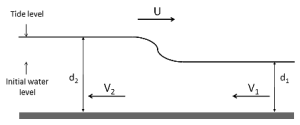Fig. 1: Tidal bore : positive translational surge. The bottom of the river is considered to be flat.

Introduction

A tidal bore is a sudden increase in the water depth of the river. This surge is of tidal origin. It results from the upstream propagation of a rising tide into estuaries and river mouths. At a given time the tidal bore represent the upstream extremity of the tidal rise. Such a situation is called a positive translational surge. This materializes in a wave or a train of waves that are around one meter high and propagate at the speed of a few meters per second. Such phenomenon occurs all around the world. The most famous bores are "pocoroca" on the Amazon river in Brazil (up to 4 meter high, propagates over 15km) and the Hang-chow bore on the Qiantang river in China (up to 9 meter high).

Even though the dynamic of tidal bores is not well known (mainly due to the complex geometry of estuaries), observations during many years allow us to come up with a few requirements for the development of the tidal bore: the bottom of the river must be relatively flat, the estuary must be convergent and the tidal amplitude must be above 6 to 9 meters. In this report I will first explain the propagation, using a quasi-steady flow analysis. Then using the perturbation to this former analysis I will try to explain the formation of such a surge and finally I will show the dynamic of the formation of the surge using a finite difference solution of the one dimensional shallow water equation.

Let us first consider that the tidal bore is already developed (we will come back to the formation step later) as in Fig. 1.

The parameters involve in the model are V1 and V2 the downstream and upstream flow velocity, d1 and d2 the downstream and upstream water depth and U the velocity of the tidal bore. In this quasi steady flow analysis, we assume that all this quantities are constant and that the shape of the hydraulic surge does not vary over time. In the reference frame of the surge, this model is similar to that of the static hydraulic jump. Then the mass conservation and the momentum conservation give the two following relations: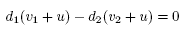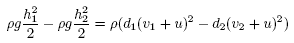To calculate the wave velocity u, v2 is eliminated from the momentum equation: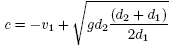Then by knowing the upstream flow velocity and the water elevation, we can deduce the propagation speed of the surge. Since c is the velocity in the reference frame of the bank, a tidal bore can only be observe if this quantity is positive. From this relation it is clear that it possible for the surge to propagate upstream only if the water depth d1 is large enough or the upstream velocity v1 is small enough.

Formation and Stability from Perturbation

Now that we have derived the steady state solution, we need to understand why it exists or in other word why it is stable. To do so one must consider small perturbations to the above solution. What happen if there is a small wave created upstream or downstream of the surge?

Let's first consider a small wave upstream. In the limit of infinitesimal small wave (small difference between d1 and d2), the velocity tends to: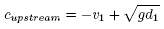It can be seen that the velocity of a given wave is always superior to the limit of small wave propagating upstream. In terms of stability it means that if a small wave is created upstream from the surge, the surge will catch up.

Now let us consider a small wave propagating downstream from the surge its velocity is: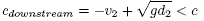So infinitesimal small wave create downstream will catch up with the front of the surge. To sum up this perturbation analysis shows that the surge is a stable phenomenon and has a soliton like propagation. Moreover as the tide continues to rise small wave will be created downstream and will stack up to the front to further increase the surge amplitude.

More Advanced Dynamic Model

The dynamic of the formation of the surge can be obtained by using the one-dimensional Korteweg-de Vries equation (KdV), one formulation of the shallow water equatation. This equation is derived from the Euler equation assuming a one dimensional flat and shallow open flow channel. The derivation also considers small wave amplitude of long wavelength and the water tension is neglected. This means that this equation is particularly well adapted to understand the formation of the surge but it might not be accurate to describe the surge once the slope became too important. In the reference frame of the flow the KdV equation is: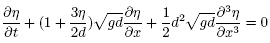Where d is the water depth at rest, η is the elevation above the rest surface d. In the limit of infinitesimal wave of long wavelength, the equation reduces to:The speed of the infinitesimal wave, in the reference frame of the flow, is the same than the one found in the first quasi steady analysis. To observe the formation of the surge, I compute this equation using a finite difference scheme. I implement it using c, the source code can be found here . In this simulation I model a channel of depth 20cm and a water elevation of 5cm that start with a slope of around 0.2%, after around 35 sec propagation the water elevation reaches a slope of around 5%. So starting from a given water elevation over a long distance a surge can take place.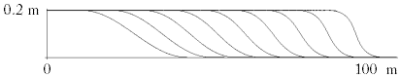Fig. 2: Surge Propagation in the reference frame of the upstream flow. From left to right, we can see the surge propagating and steepening (one plot each 5s). The last plot shows the surge after 35s.

Conclusion

Tidal Bores are phenomenon that has been observed for a long time. The complexity of its environment, especially the topography of the estuary prevents from obtaining a good quantitave understanding. However its formation and propagation can be qualitatively understood using a relatively simple one-dimensional analysis. It requires a combination of favorable condition (topography, tide amplitude, river flux) to develop. As consequence, it remains a rare phenomenon that takes place a few places in the world.

© 2007 A. Serpry. The author grants permission to copy, distribute and display this work in unaltered form, with attribution to the author, for noncommercial purposes only. All other rights, including commercial rights, are reserved to the author.

References

 H. Chason, "Mascaret, Aegir, Pororoca, Tidal Bore. Quid? Où? Quand? Comment? Pourquois?," Journal La Houille Blanche, No. 3, 103 (2005).

 H. Chason, The Hydraulics of Open Channel Flow: an Introduction, (Butterworth Heinemann, 2004).

 G. A. El, R. H. J. Grimshaw and A. M. Kamchatnov, "Analytic Model for a Weakly Dissipative Shallow-Water Undular Bore," Chaos 15, 037102 (2005).

 W. Hereman, "Shallow Water Waves and Solitary Waves", in Encyclopedia of Complexity and Systems Science, ed. by R. A. Meyers (Springer, 2008).